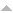## Publications

Export 5 results:
Sort by: [ Author] Title Type Year
V
Viana, L. A., Nunes A. Antão, Vicente M. da Silva, and N. M. C. Guerra The application of limit analysis to the study of the basal failure of deep excavations in clay considering the spatial distribution of soil strength. Proceedings of the 17 European Conference on Soil Mechanics and Geotechnical Engineering. Reykjavik, Islândia, 2019.
Vicente da Silva, M., and A. N. Antão. "A novel Augmented Lagrangian Approach for Limit Analysis Computations." IOP Conf. Ser.: Mater. Sci. Eng. 10. 10 (2010). AbstractWebsite

n/a

Vicente da Silva, M., and A. N. Antão. "Upper bound limit analysis with a parallel mixed finite element formulation." International Journal of Solids and Structures. 45 (2008): 5788-5804. AbstractWebsite

This paper addresses an implementation of the upper bound limit analysis theorem using a parallel mixed finite element formulation. The intrinsic characteristics of the adopted upper bound formulation proved to be suitable to adapt it to an efficient parallelization scheme. In order to illustrate the computational power provided by the new parallel processing method, accurate upper bound collapse load estimates, for 3D problems, are produced using a cluster of common PC machines.

Vicente da Silva, M., and A. N. Antão. "A non-linear programming method approach for upper bound limit analysis." International Journal for Numerical Methods in Engineering. 72 (2007): 1192-1218. AbstractWebsite

This paper presents a finite element model based on mathematical non-linear programming in order to determine upper bounds of colapse loads of a mechanical structure.The proposed formulation is derived within a kinematical approach framework, employing two simultaneous and independent field approximations for the velocity and strain rate fields. The augmented Lagrangian is used to establish the compatibility between these two fields. In this model, only continuous velocity fields are used.Uzawa's minimization algorithm is applied to determine the optimal kinematical field that minimizes the difference between external and dissipated work rate. The use of this technique allows to bypass the complexity of the non-linear aspects of the problem, since non-linearity is addressed as a set of small local subproblems of optimization for each finite element.The obtained model is quite versatile and suitable for solving a wide range of collapse problems. This paper studies 3D strut-and-tie structures, 2D plane strain/stress and 3D solid problems.

Vicente da Silva, M., and A. N. Antão. "A novel augmented Lagrangian based formulation for upper bound limit analysis." International Journal for Numerical Methods in Engineering. vol. 89.nº 12 (2012): pp. 1471-1496. Abstract

This paper describes a novel upper-bound formulation of limit analysis. This formulation is an innovative variant of an existing two-field mixed formulation based on the augmented Lagrangian method also developed by the authors. A natural approach is used to describe the deformation of each finite element. Furthermore, and in contrast to the previous formulation, two independent field approximations are now both used to define the velocity field, defined globally and at element level. It is shown that this feature allows a governing system of uncoupled linear equations to be obtained. Some numerical examples in plane strain conditions are presented in order to illustrate the current model performance. In conclusion, the potential and advantages of this new approach are discussed.